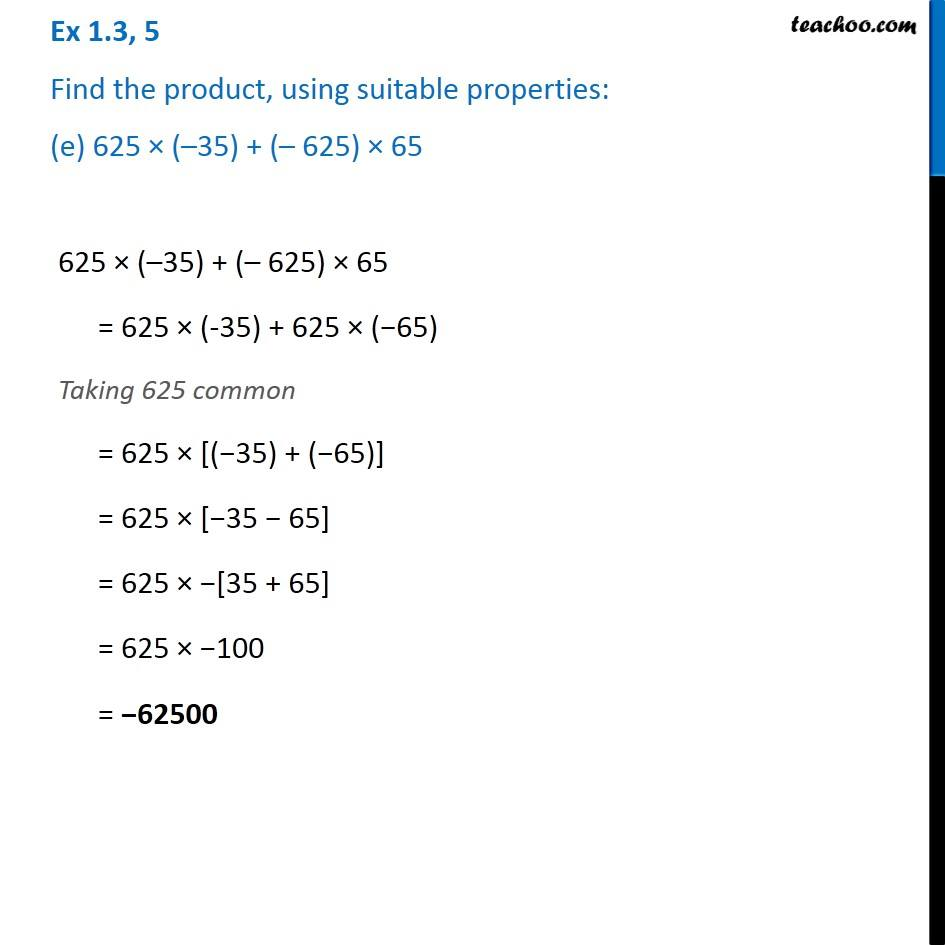1. Chapter 1 Class 7 Integers
2. Serial order wise
3. Ex 1.3

Transcript

Ex 1.3, 5 Find the product, using suitable properties: (e) 625 × (–35) + (– 625) × 65 625 × (–35) + (– 625) × 65 = 625 × (-35) + 625 × (−65) Taking 625 common = 625 × [(−35) + (−65)] = 625 × [−35 − 65] = 625 × −[35 + 65] = 625 × −100 = −62500